Test: Zeroes & Coefficients

# Test: Zeroes & Coefficients - Class 10

Test Description

## 20 Questions MCQ Test Mathematics (Maths) Class 10 - Test: Zeroes & Coefficients

Test: Zeroes & Coefficients for Class 10 2023 is part of Mathematics (Maths) Class 10 preparation. The Test: Zeroes & Coefficients questions and answers have been prepared according to the Class 10 exam syllabus.The Test: Zeroes & Coefficients MCQs are made for Class 10 2023 Exam. Find important definitions, questions, notes, meanings, examples, exercises, MCQs and online tests for Test: Zeroes & Coefficients below.
Solutions of Test: Zeroes & Coefficients questions in English are available as part of our Mathematics (Maths) Class 10 for Class 10 & Test: Zeroes & Coefficients solutions in Hindi for Mathematics (Maths) Class 10 course. Download more important topics, notes, lectures and mock test series for Class 10 Exam by signing up for free. Attempt Test: Zeroes & Coefficients | 20 questions in 20 minutes | Mock test for Class 10 preparation | Free important questions MCQ to study Mathematics (Maths) Class 10 for Class 10 Exam | Download free PDF with solutions
 1 Crore+ students have signed up on EduRev. Have you?
Test: Zeroes & Coefficients - Question 1

### If α and β are the zeroes of the polynomial 5x2 – 7x + 2, then sum of their reciprocals is:

Detailed Solution for Test: Zeroes & Coefficients - Question 1

We have 2 find (1/α + 1/β)

now 1/α + 1/β = (α + β)/ α β (taking LCM)

now by the given poly. we get

(α + β) = -b/a = 7/5

α β = c/a = 2/5

so, (α + β)/ α β = (7/5) / (2/5)

= 7/2

So, 1/α + 1/β = (α + β)/ α β = 7/2

Hence, 1/α + 1/β = 7/2

Test: Zeroes & Coefficients - Question 2

### If one zero of polynomial f(x) = (k2 + 4)x2 + 13x + 4k is reciprocal of the other, then k =

Detailed Solution for Test: Zeroes & Coefficients - Question 2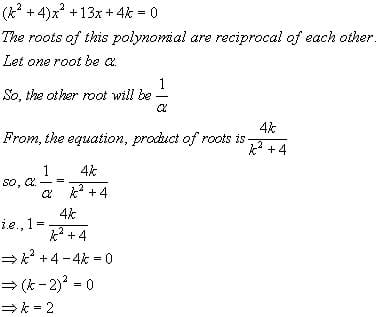Test: Zeroes & Coefficients - Question 3

### Find the quadratic polynomial whose zeros are 2 and -6

Detailed Solution for Test: Zeroes & Coefficients - Question 3

We know that quadratic equation is of the form x2-(sum of zeros)x+product of zeros
Sum of zeros=2-6=-4
Product of zeros=2*(-6)=-12
So the equation is x2+4x-12

Test: Zeroes & Coefficients - Question 4

If α, β, γ be the zeros of the polynomial p(x) such that α + β + γ = 3, αβ + βγ + γα = 10 and αβγ = -24, then p(x) is

Test: Zeroes & Coefficients - Question 5

If -√5 and √5 are the roots of the quadratic polynomial. Find the quadratic polynomial.

Detailed Solution for Test: Zeroes & Coefficients - Question 5

We have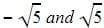as the roots which means x +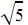and x-are the factors of the quadratic equation. Multiplying x+and x-and applying a2-bwe get the equation x2-5.

Test: Zeroes & Coefficients - Question 6

If one root of polynomial equation ax2 + bx + c = 0 be reciprocal of other, then

Detailed Solution for Test: Zeroes & Coefficients - Question 6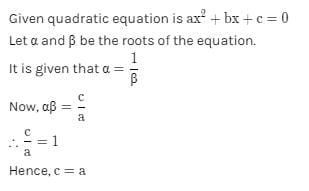Test: Zeroes & Coefficients - Question 7

If one zero of the polynomial x2 + kx + 18 is double the other zero then k = ?

Detailed Solution for Test: Zeroes & Coefficients - Question 7

Let one zero of the equation be x
Then, other would be 2x
As we know,
x × 2x = 18
x2 = 9
x = 3, -3
Case 1:
If x = 3, 2x = 6
Therefore, -k = sum of roots = 9
⇒ k = -9
Case 2:
If x = -3, 2x = -6
Therefore, -k = -9
⇒ k = 9
So, k can be 9,-9. Therefore, option 'b' is correct.

Test: Zeroes & Coefficients - Question 8

If one zero of 2x2 – 3x + k is reciprocal to the other, then the value of k is :​

Detailed Solution for Test: Zeroes & Coefficients - Question 8

Given: 2x²-3x+k .............eq 1

Let the 2 zeroes be α & 1/α

On comparing eq 1 & eq 2 we get

a=2 ,b = -3, c= k

Product of zeroes = α × 1/α = 1

Product of zeroes= c/a

1= k/ 2

K= 2
The value of k is 2.

Test: Zeroes & Coefficients - Question 9

Sum and the product of zeroes of the polynomial x2 +7x +10 is

Detailed Solution for Test: Zeroes & Coefficients - Question 9

x2 + 7x + 10 = (x + 2)(x + 5)

So, the value of x2 + 7x + 10 is zero when x + 2 = 0 or x + 5 = 0

Therefore, the zeroes of x2 + 7x + 10 are –2 and –5.

Sum of zeroes = -7 = –(Coefficient of x) / (Coefficient of x2)

Product of zeroes = 10 = Constant term / Coefficient of x2

Test: Zeroes & Coefficients - Question 10

If sum of the zeroes of the polynomial is 4 and their product is 4, then the quadratic polynomial is

Detailed Solution for Test: Zeroes & Coefficients - Question 10

Sum of zeros= α+β =4
Product of zeros=αβ=4
x2-(Sum of zeros)x+Product of zeros
=x2-4x+4

Test: Zeroes & Coefficients - Question 11

What value/s can x take in the expression k(x – 10) (x + 10) = 0 where k is any real number.

Detailed Solution for Test: Zeroes & Coefficients - Question 11

k(x – 10) (x + 10) =0
⇒ either k=0
Or x-10=0
Or x+10=0
Since we don’t know the value of k
So either x-10=0
x=10
Or x+10=0
x=-10
So values of x can be 10,-10

Test: Zeroes & Coefficients - Question 12

Find the sum and the product of zeroes of the polynomial x2 +7x +10

Detailed Solution for Test: Zeroes & Coefficients - Question 12

x2 + 7x + 10 = (x + 2)(x + 5)

So, the value of x2 + 7x + 10 is zero when x + 2 = 0 or x + 5 = 0

Therefore, the zeroes of x2 + 7x + 10 are –2 and –5.

Sum of zeroes = -7 = –(Coefficient of x) / (Coefficient of x2)

Product of zeroes = 10 = Constant term / Coefficient of x2

Test: Zeroes & Coefficients - Question 13

The sum and product of zeros of a quadratic polynomial are 2 and -15 respectively. The quadratic polynomial is

Test: Zeroes & Coefficients - Question 14

If the product of two zeros of the polynomial f(x) = 2x3 + 6x2 – 4x + 9 is 3, then its third zero is

Detailed Solution for Test: Zeroes & Coefficients - Question 14

Step-by-step explanation:

p(x) = 2x³ + 6x² – 4x + 9

Define α,β and γ

Let α,β and γ be the 3 roots

αβ = 3 (Given)

Find the products of all the zeros:

αβγ = - d/a

αβγ = - 9/2

Find the third zeros:

Given αβ = 3

3γ = -9/2

γ = -9/2 ÷ 3

γ = -3/2

The third zero is -3/2

Test: Zeroes & Coefficients - Question 15

If α, β are zeroes of the polynomial f(x) = x2 + 5x + 8, then value of (α + β) is

Detailed Solution for Test: Zeroes & Coefficients - Question 15

We have quadratic equation of the form
x- (sum of zeros) x + product of zeros, so comparing this with the given equation we have sum of zeros = -5
So, α + β = -5

Test: Zeroes & Coefficients - Question 16

If sum of the squares of zeros of the quadratic polynomial f(x) = x2 – 8x + k is 40, find the value of k.​

Detailed Solution for Test: Zeroes & Coefficients - Question 16

p (x)= x^2-8x+k
p (x)=Ax^2+Bx+C(The equation is in this form)

Let the zeroes be 'a' and 'b'

It is given that
=> a^2+b^2=40
=> (a+b)^2 - 2ab = 40 ----1

sum of zeroes
=> a+b = -B/A = -(-8)/1 = 8

Product of zeroes
=> a*b = C/A  = k

Substitute these values in the equation1 we get
=> 8^2 - 2k = 40
=> 64 - 2k = 40
=> 2k = 24
=> k = 12

Therefore the value of k is 12

Test: Zeroes & Coefficients - Question 17

If α , β are the zeroes of f(x) = px2 – 2x + 3p and α + β = αβ then the value of p is:​

Detailed Solution for Test: Zeroes & Coefficients - Question 17

The given polynomial is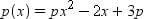Also, α and β are the zeros of p(x).

and  α + β = α × β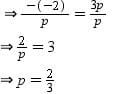Test: Zeroes & Coefficients - Question 18

If the two zeroes of the quadratic polynomial 7x2 – 15x – k are reciprocals of each other, the value of k is:​

Detailed Solution for Test: Zeroes & Coefficients - Question 18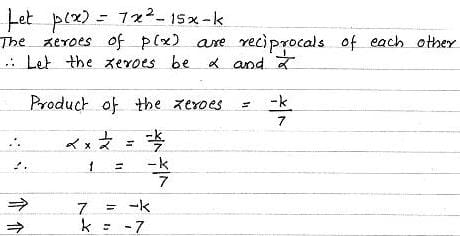Test: Zeroes & Coefficients - Question 19

The number of polynomials having zeroes -2 and 5 is:

Detailed Solution for Test: Zeroes & Coefficients - Question 19

Since the question doesn’t say that there are only 2 zeros of the polynomial we , there can be n number of polynomials which have two of its zeros as -2 and 5 .So the correct answer is more than 3.

Test: Zeroes & Coefficients - Question 20

Find the sum and the product of the zeroes of the polynomial: x2-3x-10​

Detailed Solution for Test: Zeroes & Coefficients - Question 20

X²-3x-10

x² -(5x-2x)-10

x² - 5x+2x-10

x(x-5)+2(x-5)

(x-5)(x+2)

x=5

x=-2

Sum of zeroes = α+β = 5-2 = 3
α+β = -b/a = -(-3)/1 = 3

Product of zeroes = αβ = 5*-2 = -10

αβ = c/a = -10/1 = -10

## Mathematics (Maths) Class 10

115 videos|478 docs|129 tests
Information about Test: Zeroes & Coefficients Page
In this test you can find the Exam questions for Test: Zeroes & Coefficients solved & explained in the simplest way possible. Besides giving Questions and answers for Test: Zeroes & Coefficients, EduRev gives you an ample number of Online tests for practice

## Mathematics (Maths) Class 10

115 videos|478 docs|129 tests